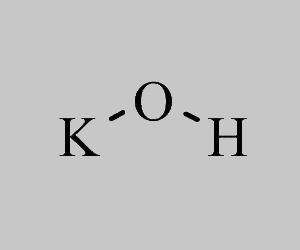# Potassium Hydroxide Formula

It is quite important to learn the potassium hydroxide formula as this chemical compound is one of the important inorganic strong bases having a wide number of applications both industrially and commercially. For example, it is used in the preparation of potassium salts, fertilizers, cleaning agents and refining petrol. Further, potassium hydroxide is basically a white solid which is highly hygroscopic. It is also sometimes called caustic potash or potassium hydrate.

## Potassium Hydroxide Chemical Formula

Potassium hydroxide has the formula written as KOH. If we break it down, hydroxide (OH) has one hydrogen and one oxygen atom with a charge of 1. Whereas potassium (K+) which is an alkali metal has a charge of +1. Therefore the equation is balanced with the charges falling into an equal ratio.

 Formula KOH Molar Mass 56.11 g mol−1 Density 2.12 g/cm3 Melting Point 360 °C Boiling Point 1,327 °C

### Potassium Hydroxide Structural Formula

The potassium hydroxide structural formula can be viewed below.Stay connected to BYJU’S to learn about the different formulas of important chemical compounds.